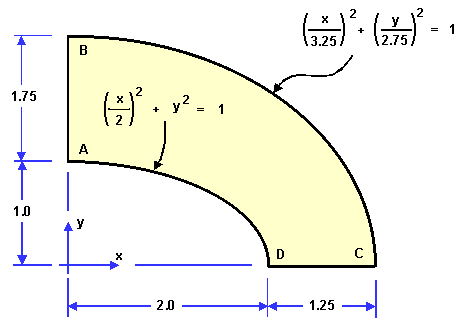# NAFEMS LE1 Benchmarck

This benchmark is extract from the Abaqus Benchmarks Manual.

## 1. Definition

We focus on the LE1 benchmarks in particular.

## 2. Running the case

The command line to run this case is

``mpirun -np 4 feelpp_toolbox_solid --case "github:{path:toolboxes/solid/NAFEMS-LE1}"``

## 3. Data files

The case data files are available in Github here

### 3.1. Geometry

The geometry is given here by :### 3.2. Boundary conditions

We set:

• $u_y = 0$ on DC

• $u_x = 0$ on AB

• $\bar{\bar{\varepsilon}}\cdot\mathbf{n}=1e^7$ on BC.

## 4. Inputs

We have the following parameters:

Table 1. Inputs
Name Value

$E$

$210\, GPa$

$\nu$

$0.3$

$\rho$

$7800\, kg/m^2$

## 5. Outputs

We want to compare the value of $\sigma_{yy}$ at the point D. The reference value is $92.7\, MPa$.

## 6. Results

The value of $\sigma_{yy}$ at the point D is $94.09\, MPa$ for $32 000$ dofs, which is $1.49%$ higher than the target.

One possibility to get a more accurate output is to use a mixed formulation, where the stress tensor would also be an unknown.